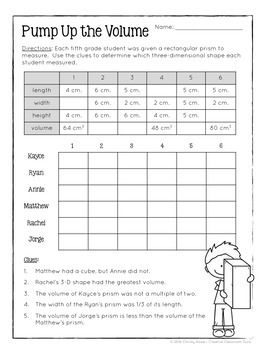digital

# Math Logic Puzzles: 5th grade Enrichment - [Digital & Printable PDF]Subject
Resource Type
Format
Zip (19 MB|28 pages)Google Apps™
Standards
\$6.50
\$6.50The Teacher-Author indicated this resource includes assets from Google Workspace (eg. docs, slides, etc.).

#### Also included in

1. This BUNDLE contains 44 Math Logic Puzzles designed to challenge and engage your high flyers and fast finishers. Your students will utilize critical thinking and problem-solving skills while building a solid understanding of essential math concepts and skills. These puzzles are available as a print
\$16.75
\$19.50
Save \$2.75

### Description

Math Logic Puzzles for 5th grade includes 15 higher-order thinking puzzles designed to challenge and engage your high flyers and fast finishers. Your students will utilize critical thinking and problem-solving skills to build a solid understanding of essential 5th-grade math concepts.

These puzzles are available as a printable PDF and a paperless version made with Google Slides™ for virtual learning.

Every activity is directly aligned with the Common Core State Standards for Math.

• Rounding and comparing decimals to the thousandths

• Adding, subtracting, multiplying, & dividing decimals

• Multi-Digit Multiplication (up to 2 digit by 5 digit)

• Multi-Digit Division (up to 2 digit by 5 digit)

• Coordinate graphing and patterns

• Adding and subtracting fractions with unlike denominators

• Multiplying and Dividing fractions

• Measuring the volume of 3-D figures

• Area & Perimeter

• Geometry (Attributes of two-dimensional shapes)

This resource includes:

• 15 No-Prep Printable Math Logic Puzzles

• Teacher Tips for Implementation

Math Logic Puzzles are great for:

• Math Centers and Stations

• Anchor Activities

• Choice Boards

• Cooperative Learning

• Independent Enrichment or Extension

• Learning Contracts

• BUILD Stations

This resource is designed for high ability 5TH-grade students. You can find logic puzzles for 3rd and 4th grade at the links below:

Save when you purchase this resource as part of the MATH LOGIC PUZZLE: BUNDLE

***************************************************************************

If you and your students enjoy these activities, you may also like the following differentiated enrichment activities:

***************************************************************************

Christy

Total Pages
28 pages
Included
Teaching Duration
N/A
Report this Resource to TpT
Reported resources will be reviewed by our team. Report this resource to let us know if this resource violates TpT’s content guidelines.

### Standards

to see state-specific standards (only available in the US).
Classify two-dimensional figures in a hierarchy based on properties.
Understand that attributes belonging to a category of two-dimensional figures also belong to all subcategories of that category. For example, all rectangles have four right angles and squares are rectangles, so all squares have four right angles.
Use a pair of perpendicular number lines, called axes, to define a coordinate system, with the intersection of the lines (the origin) arranged to coincide with the 0 on each line and a given point in the plane located by using an ordered pair of numbers, called its coordinates. Understand that the first number indicates how far to travel from the origin in the direction of one axis, and the second number indicates how far to travel in the direction of the second axis, with the convention that the names of the two axes and the coordinates correspond (e.g., 𝘹-axis and 𝘹-coordinate, 𝘺-axis and 𝘺-coordinate).
Apply and extend previous understandings of division to divide unit fractions by whole numbers and whole numbers by unit fractions.
Solve word problems involving addition and subtraction of fractions referring to the same whole, including cases of unlike denominators, e.g., by using visual fraction models or equations to represent the problem. Use benchmark fractions and number sense of fractions to estimate mentally and assess the reasonableness of answers. For example, recognize an incorrect result 2/5 + 1/2 = 3/7, by observing that 3/7 < 1/2.